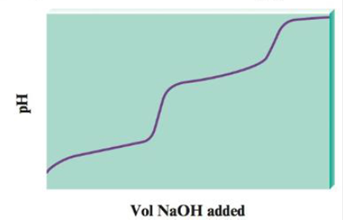### Chemistry: An Atoms First Approach

2nd Edition
Steven S. Zumdahl + 1 other
Publisher: Cengage Learning
ISBN: 9781305079243

#### Solutions

Chapter
Section### Chemistry: An Atoms First Approach

2nd Edition
Steven S. Zumdahl + 1 other
Publisher: Cengage Learning
ISBN: 9781305079243
Chapter 14, Problem 113CP
Textbook Problem
92 views

## When a diprotic acid, H2A, is titrated with NaOH, the protons on the diprotic acid are generally removed one at a time, resulting in a pH curve that has the following generic shape:a. Notice that the plot has essentially two titration curves. If the first equivalence point occurs at 100.0 mL NaOH added, what volume of NaOH added corresponds to the second equivalence point?b. For the following volumes of NaOH added, list the major species present after the OH− reacts completely.i. 0 mL NaOH addedii. between 0 and 100.0 mL NaOH addediii. 100.0 mL NaOH addediv. between 100.0 and 200.0 mL NaOH addedv. 200.0 mL NaOH addedvi. after 200.0 mL NaOH addedc. If the pH at 50.0 mL NaOH added is 4.0, and the pH at 150.0 mL NaOH added is 8.0, determine the values K a 1 , and K a 2 for the diprotic acid.

(a)

Interpretation Introduction

Interpretation: The titration curve obtained after the reaction of H2A with NaOH is given. Various questions based on the given curve are to be answered.

Concept introduction: Titration is a quantitative chemical analysis method that is used for the determination of concentration of an unknown solution. In acid base titration, the neutralization of either acid or base is done with a base or acid respectively of known concentration. This helps to determine the unknown concentration of acid or base.

When the amount of the titrant added is just sufficient for the neutralization of analyte is called equivalence point. At this point equal equivalents of both the acid and base are added.

To determine: The volume of NaOH added at the second equivalence point.

Total volume of NaOH added at the second equivalence point is 200.0ml .

### Explanation of Solution

Explanation

The reaction between H2A and OH is represented as,

H2A+OHHA+H2OHA+OHA2+H2O

The volume of NaOH added till first equivalence point is 100.0ml and is sufficient for the conversion of H2A . Now at first equivalence point, the number of moles of NaOH added is equal to the number of moles of H2A that were initially present

(b)

Interpretation Introduction

Interpretation: The titration curve obtained after the reaction of H2A with NaOH is given. Various questions based on the given curve are to be answered.

Concept introduction: Titration is a quantitative chemical analysis method that is used for the determination of concentration of an unknown solution. In acid base titration, the neutralization of either acid or base is done with a base or acid respectively of known concentration. This helps to determine the unknown concentration of acid or base.

When the amount of the titrant added is just sufficient for the neutralization of analyte is called equivalence point. At this point equal equivalents of both the acid and base are added.

(c)

Interpretation Introduction

Interpretation: The titration curve obtained after the reaction of H2A with NaOH is given. Various questions based on the given curve are to be answered.

Concept introduction: Titration is a quantitative chemical analysis method that is used for the determination of concentration of an unknown solution. In acid base titration, the neutralization of either acid or base is done with a base or acid respectively of known concentration. This helps to determine the unknown concentration of acid or base.

When the amount of the titrant added is just sufficient for the neutralization of analyte is called equivalence point. At this point equal equivalents of both the acid and base are added.

To determine: The value of Ka1 and Ka2 for the diprotic acid.

The value of Ka1 is 1.0×10-4_ and Ka2 is 1.0×10-8_

### Still sussing out bartleby?

Check out a sample textbook solution.

See a sample solution

#### The Solution to Your Study Problems

Bartleby provides explanations to thousands of textbook problems written by our experts, many with advanced degrees!

Get Started

Find more solutions based on key concepts
What do the various chromatographic separation techniques have in common?

General Chemistry - Standalone book (MindTap Course List)

Athletes should avoid frequent between-meal snacks. T F

Nutrition: Concepts and Controversies - Standalone book (MindTap Course List)

Why is this referred to as just one proposed family tree of the chordates?

Oceanography: An Invitation To Marine Science, Loose-leaf Versin

A sidewalk is to be constructed around a swimming pool that measures (10.0 0.1) m by (17.0 0.1) m. If the sid...

Physics for Scientists and Engineers, Technology Update (No access codes included)